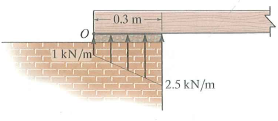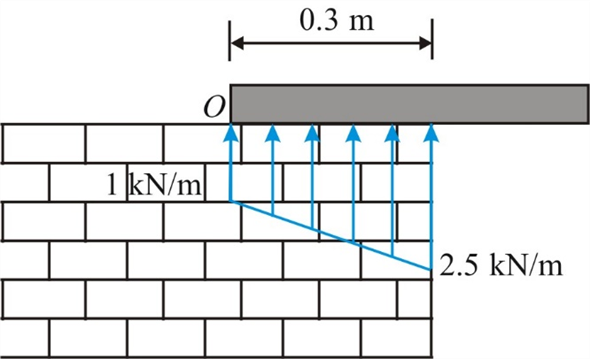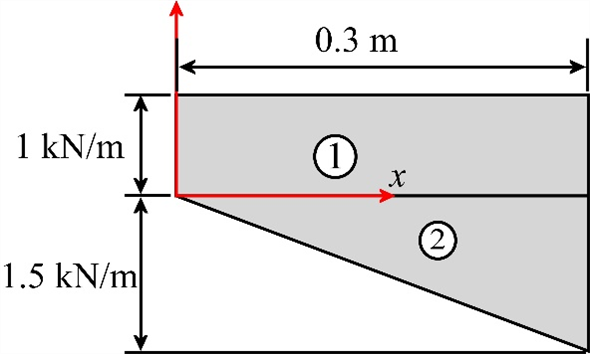Problem

# The masonry support creates the loading distribution acting on the end of the beam. Simpli...

The masonry support creates the loading distribution acting on the end of the beam. Simplify this load to a single resultant force and specify its location measured from point O.#### Step-by-Step Solution

Solution 1

Draw the free body diagram ,Calculate the equivalent resultant force of the distributed load

\begin{aligned} F_{R} &=(1 \times 0.3)+\left(\frac{1}{2} \times 0.3 \times 1.5\right) \\ &=0.3+0.225 \\ &=0.525 \mathrm{kN} \end{aligned}

Therefore, the resultant force is $$0.525 \mathrm{kN}$$

Consider the following figure.The resultant force will always acts at the centroid of the distributed area. Therefore, find location

of the resultant force acting from point $$O$$ as follows:

\begin{aligned} \bar{x} &=\frac{\sum A x}{\sum A} \\ &=\frac{A_{1} x_{1}+A_{2} x_{2}}{A_{1}+A_{2}} \\ &=\frac{(1 \times 0.3)\left(\frac{0.3}{2}\right)+\left(\frac{1}{2} \times 0.3 \times 1.5\right)\left(\frac{2}{3} \times 0.3\right)}{(1 \times 0.3)+\left(\frac{1}{2} \times 0.3 \times 1.5\right)} \\ &=\frac{0.045+0.045}{0.3+0.225} \\ \bar{x} &=0.1714 \mathrm{~m} \end{aligned}

Therefore, the location of the force from point $$O$$ is $$0.1714 \mathrm{~m}$$.